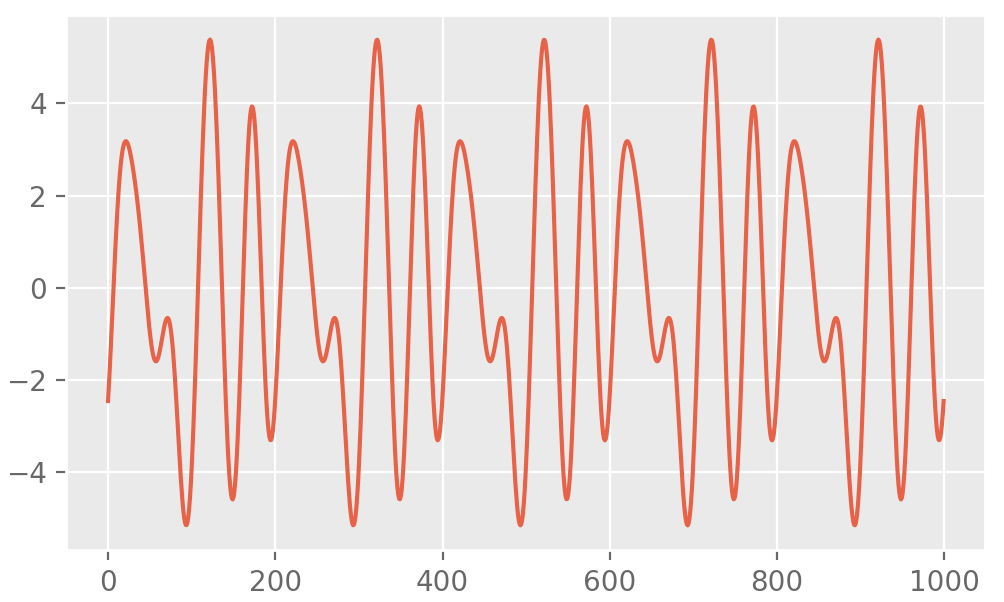# Using einsum for vectorizing matrix ops

An old theoretical physics course that I took introduced me to the wonders of the Einstein summation convention. I had to vectorize some matrix operations recently for multivariate normal liklihood calculations, $$X * X^T$$ and $$Y * X$$, on a large list of matrices (shape $$(n, k, k)$$). Using einsum for this gives a massive performance boost.

# Efficient inverse-matrix multiplication

This uses a cholesky decomposition to calculate the inverse.

$L^T / (L / y) = (LL^T)^{-1}y$

# Efficient Inference of GP Covariances

Sometimes, it is easier to do parameter inference in the frequency domain. One can use SymPy (or another symbolic computation program) to get the theoretical spectrum of a GP (using a Fourier transform of the covariance function - see the Wiener-Khinchin theorem) and we use the Whittle likelihood to fit the parameters of the process using the spectrum. Similar ideas can be found in William Wilkinson’s papers and Turner and Sahani (2014).

# Sampling Periodic GPs using the State Space Representation

The representation is due to Solin & Sarkka (2014).

Python Code# Fast Toeplitz Matrix-Vector Products and Solving

Circulant matrix-vector products can be fast due to the way circulant matrices can be decomposed using their fourier transforms. Toeplitz matrices can be ‘embedded’ into a circulant matrix and their matrix-vector products can be computed efficiently too.

Main reference:

J. Dongarra, P. Koev, and X. Li, “Matrix-Vector and Matrix-Matrix Multiplications”. In Z. Bai, J. Demmel, J. Dongarra, A. Ruhe, and H. van der Vorst, editors. “Templates for the Solution of Algebraic Eigenvalue Problems: A Practical Guide”. SIAM, Philadelphia, 2000. Available online.

This can then be used to compute multivariate normal log-densities quickly. Furthermore, this multiplication can then be used in conjugate gradient solvers to efficiently compute inverse-matrix-vector products in $$O(n \log n)$$ time and $$O(n)$$ space.

I’ve contributed a function to scipy - scipy.linalg.matmul_toeplitz that does this computation. The source can be viewed here.

Toeplitz matrices can be inverted in quadratic time using the Levinson algorithm, that also has the capability of returning a determinant as a side product using no more computation (as I understand).

# Toeplitz Matrix Cholesky Decomposition

I got the toeplitz_cholesky library from here and compiled it (ctypes is used to load this into python). I believe that the following algorithm calculates the cholesky decomposition in $$O(n^2)$$ time.

C/Python Code

# Using einsum for vectorizing matrix ops

### Gaussian Processes in MGCV

I lay out the canonical GP interpretation of MGCV’s GAM parameters here. Prof. Wood updated the package with stationary GP smooths after a request. Running through the predict.gam source code in a debugger, the computation of predictions appears to be as follows:

## Snowflake GP

### Photogrammetry

I wanted to see how easy it was to do photogrammetry (create 3d models using photos) using PyTorch3D by Facebook AI Research.

### Dead Code & Syntax Trees

This post was motivated by some R code that I came across (over a thousand lines of it) with a bunch of if-statements that were never called. I wanted an automatic way to get a minimal reproducing example of a test from this file. While reading about how to do this, I came across Dead Code Elimination, which kills unused and unreachable code and variables as an example.

## 2020

### Astrophotography

I used to do a fair bit of astrophotography in university - it’s harder to find good skies now living in the city. Here are some of my old pictures. I’ve kept making rookie mistakes (too much ISO, not much exposure time, using a slow lens, bad stacking, …), for that I apologize!

### Probabilistic PCA

I’ve been reading about PPCA, and this post summarizes my understanding of it. I took a lot of this from Pattern Recognition and Machine Learning by Bishop.

### Spotify Data Exploration

The main objective of this post was just to write about my typical workflow and views. The structure of this data is also outside my immediate domain so I thought it’d be fun to write up a small diary working with the data.

### Random Stuff

For dealing with road/city networks, refer to Geoff Boeing’s blog and his amazing python package OSMnx. Go to Shapely for manipulation of line segments and other objects in python, networkx for networks in python and igraph for networks in R.

### Morphing with GPs

The main aim here was to morph space inside a square but such that the transformation preserves some kind of ordering of the points. I wanted to use it to generate some random graphs on a flat surface and introduce spatial deformation to make the graphs more interesting.

### Speech Synthesis

The initial aim here was to model speech samples as realizations of a Gaussian process with some appropriate covariance function, by conditioning on the spectrogram. I fit a spectral mixture kernel to segments of audio data and concatenated the segments to obtain the full waveform.

Consider: